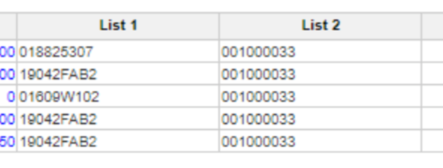# Multiple SUM&LOOKUP splitting workaroundHi,

I am trying to split multiple SUM&LOOKUP's used in a lineitem in a  module in order to optimize the model performance. But while splitting the SUM & LOOKUP into different line items in separate modules (dimensioned by lists used in SUM&LOOKUP)  , the result is not coming as expected. Since the size of lists is quite huge, i cannot even combine the lists being used in the SUM&LOOKUP  as part of one module and then aggregate the value to final module. I know its not best practice to use multiple SUM&LOOKUP together, but the requirement is such that we have to sum the transaction value based on multiple attributes . Is there any workaround for the same where more than 3 SUM&LOOKUP are being used in a single line item

Khushboo

Tagged:

•Can you please provide a little more information?  Ideally, you would create a Calc Module that just does the sum piece of the attributes needing to be summed.  Then, you can do a lookup to that module in order to get the desired information.  Again, if you can provide more information, maybe we can be of more help.

Rob

•Thanks @rob_marshall

I have attached screenshot of SUM&LOOKUP i am trying to do using Calc modules as you have mentioned already. But this approach seems to work only if we have one attribute to do SUM&LOOKUP on. In case of multiple attributes, the result doesnt seem to look correct.

Below is how the splitting of lineitems is done in the Final module:

Value: Line item which need to be aggregated based on List 1 and List 2

Aggregate level 1: 'Sum Calc 1'. 'Aggregate 1'[LOOKUP:List1]

Final Aggregation: 'Sum Calc 2'. 'Aggregate 2'[LOOKUP:List2]

Let me know if you need more details

•See if this helps

1. Create a module (call it Module A) dimensioned by List 1 and List 2 and use below formula in Line item (call it X)

X = Final Module.Value[SUM: Final Module.List 1, SUM: Final Module. List 2]

2. In your Final Module Expected Value Line item  use below formula

Expected Value = Module A.X[LOOKUP: List 1, LOOKUP: List 2]

Misbah

•This would give me correct result, however issue in my case is the size of lists being used is really huge (~ million items in each list). And combining them in a separate module is increasing the model size by 10 GB.

•Ah I see!! If you ask me I would always give paramount importance to the performance. End users don’t see or don’t

know the size impact but yes you will have to take a judicious call in this situation and see if consuming this amount of space is actually improving your performance. Also in Hypermodel world it becomes more important than ever to follow best practices and not worry much about space.  Will let @rob_marshall  speak more on this

Cheers

Misbah

•So, each one of those lists comprise of a million+ members, is that correct?  Combining those into one module would be expensive from a space perspective.  A couple of questions:

• is there a relationship between the two lists?  As in, is List 2 an attribute of List 1?
• what did the original SUM/LOOKUP formula look like, or is that in the Final Module picture?

Thanks,

Rob

•Yes correct, there are million+ items in each of these list. To answer your questions,

1. Apparently there is no relation between List 1 and List 2. However, both of these are as attributes in the Final Module.

2. Original SUM&LOOKUP was: Value[SUM:List 1,LOOKUP: List 1, SUM: List 2, LOOKUP: List 2]

•Do the members in List 1 span multiple members in List 2?  Meaning can 19042FAB2 have different List 2 members other than 001000033 or is 19042FAB2 always tied to 001000033?If the answer is yes, you can create a list (not really a fan of this but it beats the 10 GB space issue) where you find the first occurrence of List 1 and List 2 concatenated together.  Then, in this module, you create a line item doing a FindItem(New List, List 1_List2).  In a new Calc module, you sum the data off the Finditem() member.  This way, you will get the correct numbers and it will not be so crazy sparse.

Rob

•Yes this approach works for me. I am concatenating items from both lists and doing SUM on line item (module based on concatenated items list) and then doing lookup on another lineitem (Final module).

Thanks!